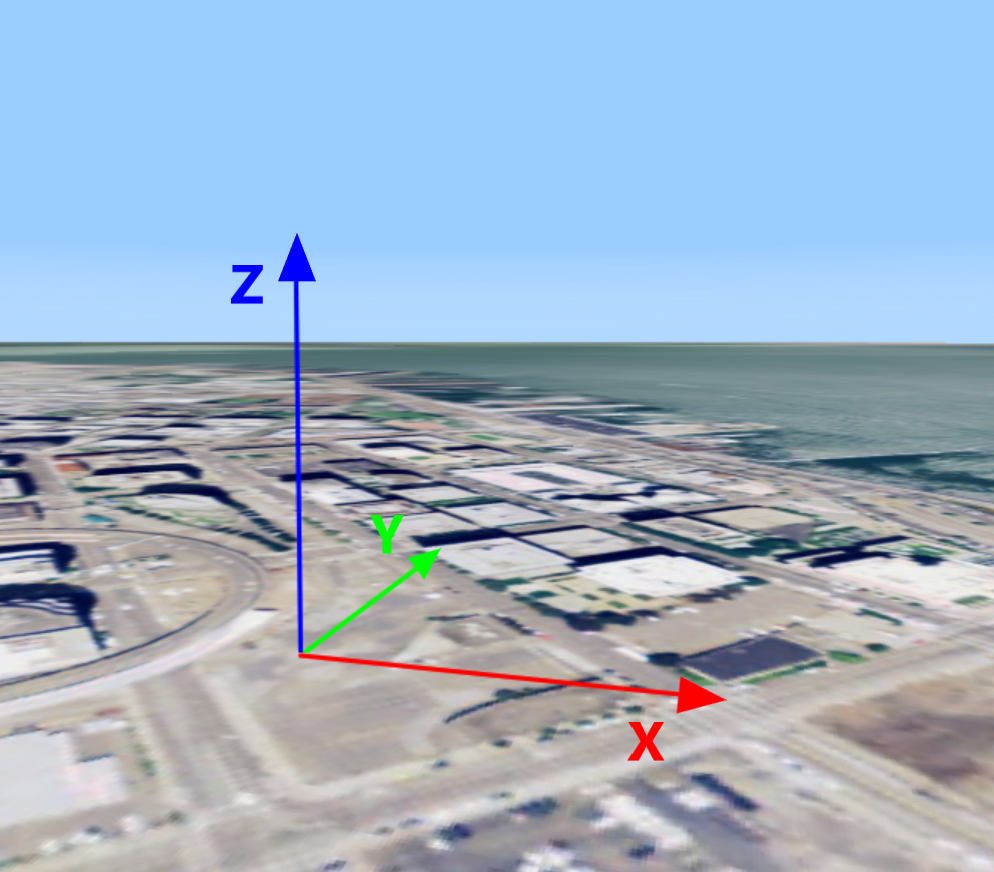## What is a topocentric reference?

A topocentric reference is a local, Cartesian coordinate reference that you can place anywhere in the world. It’s defined by a georeferenced point that specifies the origin of its coordinate system.

A topocentric reference has these properties:

• The origin is defined by a geospatial point. This point can be anywhere in the world.

• The X-axis points East, the Y-axis points North, and the Z-axis points upwards.

• The XY-plane is tangential to the Earth ellipsoid, or symmetric with the tangential plane if the origin is not a point on the ellipsoid.

• The Z-axis is perpendicular to the XY-plane and denotes height.

• The unit of measurement on all axes is meter.Figure 1. Axes of a topocentric reference, depicted on a 3D LuciadRIA map. The X-axis points East, the Y-axis points North, and the Z-axis points upwards.

## Creating a topocentric reference

Program: Creating a topocentric reference illustrates how you define a custom origin point, and get a topocentric reference for that point from the `ReferenceProvider`.

Program: Creating a topocentric reference
``````const wgs84 = getReference("CRS:84");
const originLLH = createPoint(wgs84, [-122.39318, 37.78975, 0]);
const reference = createTopocentricReference({name: "TOPO", origin: originLLH});``````

## Transforming points to and from a topocentric reference

Using LuciadRIA’s transformations API, you can transform point coordinates between topocentric references and any other geospatial coordinate reference. For example, you could transform WGS 84 point coordinates to local point coordinates:

Program: Creating a transformation from WGS 84 to a topocentric reference
``````const wgs84 = getReference("CRS:84");
const originLLH = createPoint(wgs84, [-122.39318, 37.78975, 0]);
const localReference = createTopocentricReference({origin: originLLH});

const wgs84ToLocal = createTransformation(wgs84, localReference);

const lonLatCoordinate = createPoint(wgs84, [12, 34, 56]);
const localCoordinate = wgs84ToLocal.transform(lonLatCoordinate);``````